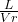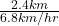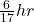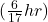## Q1: A runner is jogging in a straight line at a steady vr= 6.8 km/hr. When the runner is L= 2.4 km from the finish line, a bird begins flyin

Question

Q1: A runner is jogging in a straight line at a steady vr= 6.8 km/hr. When the runner is L= 2.4 km from the finish line, a bird begins flying straight from the runner to the finish line at vb= 13.6 km/hr (2 times as fast as the runner). When the bird reaches the finish line, it turns around and flies directly back to the runner. What cumulative distance does the bird travel? Even though the bird is a dodo, assume that it occupies only one point in space (a “zero” length bird), travels in a straight line, and that it can turn without loss of speed. Answer in units of km. Q2: After this first encounter, the bird then turns around and flies from the runner back to the finish line, turns around again and flies back to the runner. The bird repeats the back and forth trips until the runner reaches the finish line. How far does the bird travel from the beginning (including the distance traveled to the first encounter)? Answer in units of km.

in progress 0
2 months 2021-07-28T07:13:34+00:00 1 Answers 0 views 0

Q1: 3.2km

Q2: 4.8K

Explanation:

Q1:

So db is the distance of bird, and dr is the distance of runner

db = 2vr  and the distance of bird is going to be 2 times greater than the runner.

formulas: db = 2vr & db = 2dr

1. db = 2dr
2. L + (L – x) = 2x
3. 2L – x = 2x
4. 2L = 3x
5. x =L

Insert it in x =L(2.4km) = 1.6km

Now we use formula db = 2dr

1. db = 2L – x
2. db = 2(2.4km) – 1.6km
3. db = 3.2km

Q2:

Formulas: Vr = L /Δt & Vb = db/Δt

1. Vr = L/ Δt ⇒ Δt =2.3.(Km cancel each other)

1. Vb = db/Δt ⇒ db = VbΔt
2. 13.6km/hr3. 4.8km

(hr cancel each other)

Hope it helps you 🙂